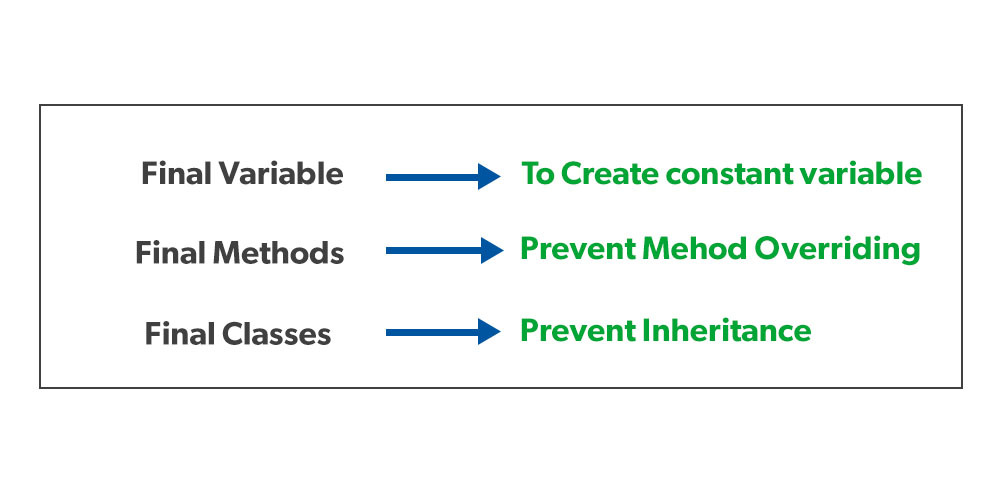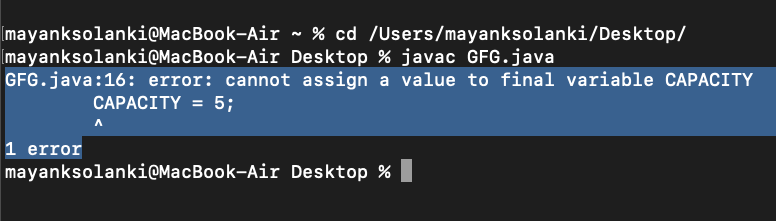# final Keyword in Java

• Difficulty Level : Easy
• Last Updated : 25 Jun, 2022

final keyword is used in different contexts. First of all, final is a non-access modifier applicable only to a variable, a method, or a class. The following are different contexts where final is used.## Final Variables

When a variable is declared with the final keyword, its value can’t be modified, essentially, a constant. This also means that you must initialize a final variable. If the final variable is a reference, this means that the variable cannot be re-bound to reference another object, but the internal state of the object pointed by that reference variable can be changed i.e. you can add or remove elements from the final array or final collection. It is good practice to represent final variables in all uppercase, using underscore to separate words.

Illustration:

```final int THRESHOLD = 5;
// Final variable```
```final int THRESHOLD;
// Blank final variable```
```static final double PI = 3.141592653589793;
// Final static variable PI```
```static final double PI;
// Blank final static  variable```

### Initializing a final Variable

We must initialize a final variable, otherwise, the compiler will throw a compile-time error. A final variable can only be initialized once, either via an initializer or an assignment statement. There are three ways to initialize a final variable:

1. You can initialize a final variable when it is declared. This approach is the most common. A final variable is called a blank final variable if it is not initialized while declaration. Below are the two ways to initialize a blank final variable.
2. A blank final variable can be initialized inside an instance-initializer block or inside the constructor. If you have more than one constructor in your class then it must be initialized in all of them, otherwise, a compile-time error will be thrown.
3. A blank final static variable can be initialized inside a static block.

Let us see these two different ways of initializing a final variable:

## Java

 `// Java Program to demonstrate Different``// Ways of Initializing a final Variable`` ` `// Main class ``class` `GFG {``   ` `    ``// a final variable``    ``// direct initialize``    ``final` `int` `THRESHOLD = ``5``;``     ` `    ``// a blank final variable``    ``final` `int` `CAPACITY;``     ` `    ``// another blank final variable``    ``final` `int`  `MINIMUM;``     ` `    ``// a final static variable PI``    ``// direct initialize``    ``static` `final` `double` `PI = ``3.141592653589793``;``     ` `    ``// a  blank final static  variable``    ``static` `final` `double` `EULERCONSTANT;``     ` `    ``// instance initializer block for ``    ``// initializing CAPACITY``    ``{``        ``CAPACITY = ``25``;``    ``}``     ` `    ``// static initializer block for ``    ``// initializing EULERCONSTANT``    ``static``{``        ``EULERCONSTANT = ``2.3``;``    ``}``     ` `    ``// constructor for initializing MINIMUM``    ``// Note that if there are more than one``    ``// constructor, you must initialize MINIMUM``    ``// in them also``    ``public` `GFG() ``    ``{``        ``MINIMUM = -``1``;``    ``}``         ` `}`

Geeks there was no main method in the above code as it was simply for illustration purposes to get a better understanding in order to draw conclusions:

Observation 1: When to use a final variable?

The only difference between a normal variable and a final variable is that we can re-assign the value to a normal variable but we cannot change the value of a final variable once assigned. Hence final variables must be used only for the values that we want to remain constant throughout the execution of the program.

Observation 2: Reference final variable?

When a final variable is a reference to an object, then this final variable is called the reference final variable. For example, a final StringBuffer variable looks defined below as follows:

`final StringBuffer sb;`

As we all know that a final variable cannot be re-assign. But in the case of a reference final variable, the internal state of the object pointed by that reference variable can be changed. Note that this is not re-assigning. This property of final is called non-transitivity. To understand what is meant by the internal state of the object as shown in the below example as follows:

Example 1:

## Java

 `// Java Program to demonstrate``// Reference of Final Variable`` ` `// Main class``class` `GFG {`` ` `    ``// Main driver method``    ``public` `static` `void` `main(String[] args)``    ``{``        ``// Creating sn object of StringBuilder class``        ``// Final reference variable``        ``final` `StringBuilder sb = ``new` `StringBuilder(``"Geeks"``);`` ` `        ``// Printing the element in StringBuilder object``        ``System.out.println(sb);`` ` `        ``// changing internal state of object reference by``        ``//  final reference variable sb``        ``sb.append(``"ForGeeks"``);`` ` `        ``// Again printing the element in StringBuilder``        ``// object after appending above element in it``        ``System.out.println(sb);``    ``}``}`

Output

```Geeks
GeeksForGeeks```

The non-transitivity property also applies to arrays, because arrays are objects in Java. Arrays with the final keyword are also called final arrays.

Note: As discussed above, a final variable cannot be reassign, doing it will throw compile-time error.

Example 2:

## Java

 `// Java Program to Demonstrate Re-assigning``// Final Variable will throw Compile-time Error`` ` `// Main class``class` `GFG {`` ` `    ``// Declaring and customly initializing``    ``// static final variable``    ``static` `final` `int` `CAPACITY = ``4``;`` ` `    ``// Main driver method``    ``public` `static` `void` `main(String args[])``    ``{`` ` `        ``// Re-assigning final variable``        ``// will throw compile-time error``        ``CAPACITY = ``5``;``    ``}``}`

Output:Remember: When a final variable is created inside a method/constructor/block, it is called local final variable, and it must initialize once where it is created. See below program for local final variable.

Example:

## Java

 `// Java program to demonstrate``// local final variable`` ` `// Main class``class` `GFG {`` ` `    ``// Main driver method``    ``public` `static` `void` `main(String args[])``    ``{``        ``// Declaring local final variable``        ``final` `int` `i;`` ` `        ``// Now initializing it with integer value``        ``i = ``20``;`` ` `        ``// Printing the value on console``        ``System.out.println(i);``    ``}``}`

Output

`20`

Remember the below key points as perceived before moving forward as listed below as follows:

1. Note the difference between C++ const variables and Java final variables. const variables in C++ must be assigned a value when declared. For final variables in Java, it is not necessary as we see in the above examples. A final variable can be assigned value later, but only once.
2. final with foreach loop: final with for-each statement is a legal statement.

Example:

## Java

 `// Java Program to demonstrate Final``// with for-each Statement`` ` `// Main class``class` `GFG {`` ` `    ``// Main driver method``    ``public` `static` `void` `main(String[] args)``    ``{`` ` `        ``// Declaring and initializing``        ``// custom integer array``        ``int` `arr[] = { ``1``, ``2``, ``3` `};`` ` `        ``// final with for-each statement``        ``// legal statement``        ``for` `(``final` `int` `i : arr)``            ``System.out.print(i + ``" "``);``    ``}``}`

Output

`1 2 3 `

Output explanation: Since the “i” variable goes out of scope with each iteration of the loop, it is actually re-declaration each iteration, allowing the same token (i.e. i) to be used to represent multiple variables.

### Final classes

When a class is declared with final keyword, it is called a final class. A final class cannot be extended(inherited).

There are two uses of a final class:

Usage 1: One is definitely to prevent inheritance, as final classes cannot be extended. For example, all Wrapper Classes like Integer, Float, etc. are final classes. We can not extend them.

```final class A
{
// methods and fields
}
// The following class is illegal
class B extends A
{
// COMPILE-ERROR! Can't subclass A
}```

Usage 2: The other use of final with classes is to create an immutable class like the predefined String class. One can not make a class immutable without making it final.

### Final Methods

When a method is declared with final keyword, it is called a final method. A final method cannot be overridden. The Object class does this—a number of its methods are final. We must declare methods with the final keyword for which we are required to follow the same implementation throughout all the derived classes.

Illustration: Final keyword with a method

```class A
{
final void m1()
{
System.out.println("This is a final method.");
}
}

class B extends A
{
void m1()
{
// Compile-error! We can not override
System.out.println("Illegal!");
}
}```

For more examples and behavior of final methods and final classes, please see Using final with inheritance. Please see the abstract in java article for differences between the final and abstract.
Related Interview Question(Important): Difference between final, finally, and finalize in Java